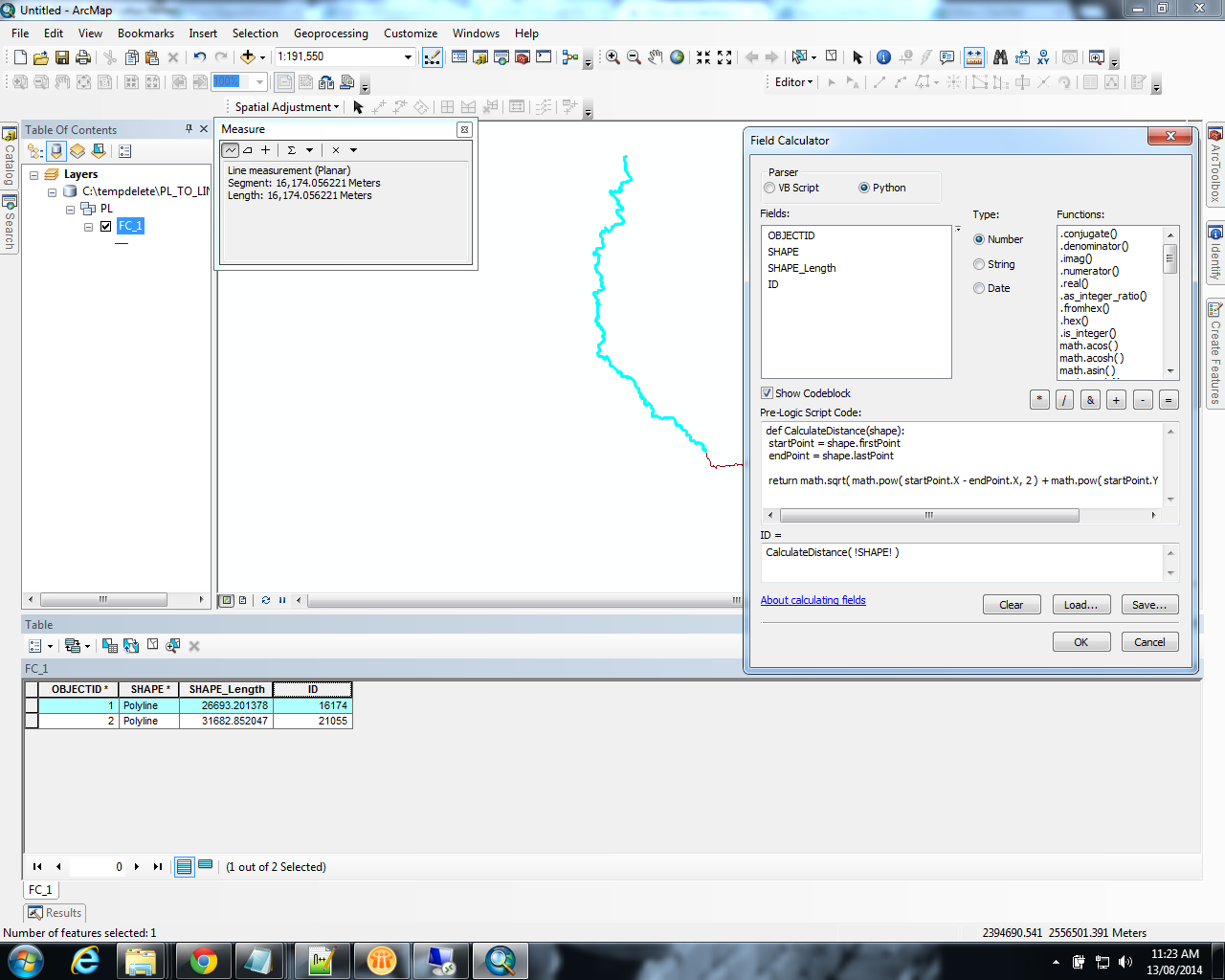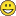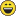# Distance between start- and end-points

8997
8
08-12-2014 04:22 PMOccasional Contributor III

Hi,

How do I measure the distance between the start and end points of a line?  So not the length of the line itself, but the straight line distance between the two points?

Thanks,
Mike.

1 Solution

Accepted SolutionsRegular Contributor

Hi Mike,

Below function lets you calculate the shortest distance between start and end point of a line as an attribute column in arcmap.

def CalculateDistance(shape):

startPoint = shape.firstPoint

endPoint = shape.lastPoint

return math.sqrt( math.pow( startPoint.X - endPoint.X, 2 ) + math.pow( startPoint.Y - endPoint.Y, 2 ))8 RepliesRegular Contributor

If you have the coordinates of the points (P1, P2) then.

d = Math.sqrt(Math.Pow(P1.x - p2.X, 2) + Math.Pow(P1.y - P2.y, 2))Regular Contributor

If you want to calculate this for a line feature class you can follow these steps - not sure if there is a simpler way but it works1. Make sure you have a unique field that identifies each line, for example copy the object id into a new field called LineID.
2. Add the following fields to your data: StartX, StartY, EndX, EndY,  LineDist (all double field type)
3. Open the attribute table, right-click on the new field headers and use the Calculate Geometry option to populate the fields (X Coordinate of Line Start, Y Coordinate of Line Start, etc)
4. From the attribute table Export the data as PointData and add it to the map (this makes the next step easier).
7. When the layer is added, right click on it and export the point feature class as StartPoints.
8. Repeat steps 5-7 to create the EndPoints feature class.
9. Use the Merge tool to combine StartPoints and EndPoints into a single AllPoints feature class.
10. Use the Points to Line tool to create lines from your points. Select LinePoints as your input, specify an output feature class and make sure to select LineID as your Line Field parameter.

Now you should have a new feature class with straight lines between your original start and end points. Open the attribute table and the lengths are all there.

You can join this data back to your original line feature class using the LineID and calculate LineDist = Shape_Length (from the join data).Occasional Contributor III

OK I see how that worksI'm hoping for something with fewer steps, however if it comes to it I can use this.

Cheers,

Mike.Occasional Contributor III
 Riyas Deen wrote: If you have the coordinates of the points (P1, P2) then. d = Math.sqrt(Math.Pow(P1.x - p2.X, 2) + Math.Pow(P1.y - P2.y, 2))

I'm sorry, but where do I input that?

Cheers,

Mike.Regular Contributor

Hi Mike,

Below function lets you calculate the shortest distance between start and end point of a line as an attribute column in arcmap.

def CalculateDistance(shape):

startPoint = shape.firstPoint

endPoint = shape.lastPoint

return math.sqrt( math.pow( startPoint.X - endPoint.X, 2 ) + math.pow( startPoint.Y - endPoint.Y, 2 ))Occasional Contributor III

thank you, that does exactly what I'm afterMuch appreciated,

Mike.Regular Contributor

Very nice - this works great and is very simple.

The results are identical to my long-winded process.byMVP Honored Contributor

Just to add, you can avoid doing the math by hand using arcpy's PointGeomtry objects and distanceTo().

```def CalculateDistance(shape):
return arcpy.PointGeometry(shape.firstPoint).distanceTo(arcpy.PointGeometry(shape.lastPoint))
```